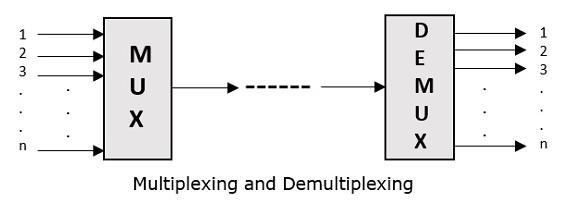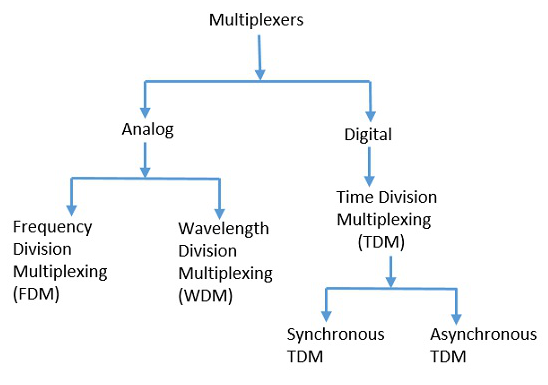# Principles of Communication - Multiplexing

Multiplexing is the process of combining multiple signals into one signal, over a shared medium.

• The process is called as analog multiplexing if these signals are analog in nature.

• If digital signals are multiplexed, it is called as digital multiplexing.

Multiplexing was first developed in telephony. A number of signals were combined to send through a single cable. The process of multiplexing divides a communication channel into several number of logical channels, allotting each one for a different message signal or a data stream to be transferred. The device that does multiplexing, can be called as a MUX.

The reverse process, i.e., extracting the number of channels from one, which is done at the receiver is called as demultiplexing. The device which does demultiplexing is called as DEMUX.

The following figures illustrates the concept of MUX and DEMUX. Their primary use is in the field of communications.## Types of Multiplexers

There are mainly two types of multiplexers, namely analog and digital. They are further divided into FDM, WDM, and TDM. The following figure gives a detailed idea about this classification.There are many types of multiplexing techniques. Of them all, we have the main types with general classification, mentioned in the above figure. Let us take a look at them individually.

## Analog Multiplexing

The analog multiplexing techniques involve signals which are analog in nature. The analog signals are multiplexed according to their frequency (FDM) or wavelength (WDM).

### Frequency Division Multiplexing

In analog multiplexing, the most used technique is Frequency Division Multiplexing (FDM). This technique uses various frequencies to combine streams of data, for sending them on a communication medium, as a single signal.

Example − A traditional television transmitter, which sends a number of channels through a single cable uses FDM.

### Wavelength Division Multiplexing

Wavelength Division multiplexing (WDM) is an analog technique, in which many data streams of different wavelengths are transmitted in the light spectrum. If the wavelength increases, the frequency of the signal decreases. A prism which can turn different wavelengths into a single line, can be used at the output of MUX and input of DEMUX.

Example − Optical fiber Communications use the WDM technique, to merge different wavelengths into a single light for the communication.

## Digital Multiplexing

The term digital represents the discrete bits of information. Hence, the available data is in the form of frames or packets, which are discrete.

### Time Division Multiplexing (TDM)

In TDM, the time frame is divided into slots. This technique is used to transmit a signal over a single communication channel, by allotting one slot for each message.

Of all the types of TDM, the main ones are Synchronous and Asynchronous TDM.

### Synchronous TDM

In Synchronous TDM, the input is connected to a frame. If there are ‘n’ number of connections, then the frame is divided into ‘n’ time slots. One slot is allocated for each input line.

In this technique, the sampling rate is common for all signals and hence the same clock input is given. The MUX allocates the same slot to each device at all times.

### Asynchronous TDM

In Asynchronous TDM, the sampling rate is different for each of the signals and a common clock is not required. If the allotted device, for a time slot transmits nothing and sits idle, then that slot is allotted to another device, unlike synchronous.

This type of TDM is used in Asynchronous transfer mode networks.

## Demultiplexer

Demultiplexers are used to connect a single source to multiple destinations. This process is the reverse of multiplexing. As mentioned previously, it is used mostly at the receivers. DEMUX has many applications. It is used in receivers in the communication systems. It is used in arithmetic and logical unit in computers to supply power and to pass on communication, etc.

Demultiplexers are used as serial to parallel converters. The serial data is given as input to DEMUX at regular interval and a counter is attached to it to control the output of the demultiplexer.

Both the multiplexers and demultiplexers play an important role in communication systems, both at the transmitter and receiver sections.